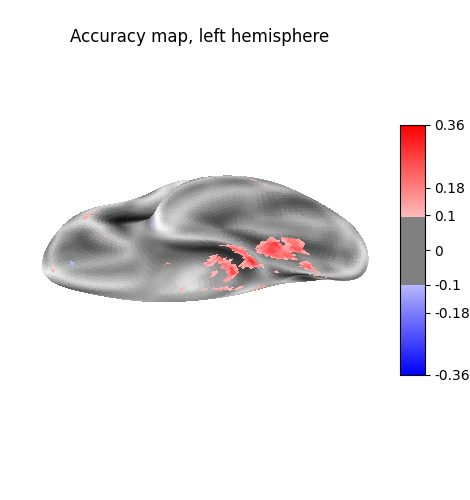# Cortical surface-based searchlight decoding#

This is a demo for surface-based searchlight decoding, as described in: Chen, Y., Namburi, P., Elliott, L.T., Heinzle, J., Soon, C.S., Chee, M.W.L., and Haynes, J.-D. (2011). Cortical surface-based searchlight decoding. NeuroImage 56, 582–592.

```import pandas as pd

from nilearn import datasets

# We fetch 2nd subject from haxby datasets (which is default)
haxby_dataset = datasets.fetch_haxby()

fmri_filename = haxby_dataset.func
y = labels["labels"]
session = labels["chunks"]
```

## Restrict to faces and houses#

```from nilearn.image import index_img

```

## Surface bold response#

```from sklearn import neighbors

from nilearn import datasets, surface

# Fetch a coarse surface of the left hemisphere only for speed
fsaverage = datasets.fetch_surf_fsaverage(mesh="fsaverage5")
hemi = "left"

# Average voxels 5 mm close to the 3d pial surface
pial_mesh = fsaverage[f"pial_{hemi}"]

# To define the :term:`BOLD` responses
# to be included within each searchlight "sphere"
# we define an adjacency matrix based on the inflated surface vertices such
# that nearby surfaces are concatenated within the same searchlight.

infl_mesh = fsaverage[f"infl_{hemi}"]
```

## Searchlight computation#

```from sklearn.linear_model import RidgeClassifier
from sklearn.model_selection import KFold
from sklearn.pipeline import make_pipeline
from sklearn.preprocessing import StandardScaler

from nilearn.decoding.searchlight import search_light

# Simple linear estimator preceded by a normalization step
estimator = make_pipeline(StandardScaler(), RidgeClassifier(alpha=10.0))

# Define cross-validation scheme
cv = KFold(n_splits=3, shuffle=False)

# Cross-validated search light
scores = search_light(X, y, estimator, adjacency, cv=cv, n_jobs=1)
```

## Visualization#

```from nilearn import plotting

chance = 0.5
plotting.plot_surf_stat_map(
infl_mesh,
scores - chance,
view="medial",
colorbar=True,
threshold=0.1,
bg_map=fsaverage[f"sulc_{hemi}"],
title="Accuracy map, left hemisphere",
)
plotting.show()
```Total running time of the script: (2 minutes 1.955 seconds)

Estimated memory usage: 916 MB

Gallery generated by Sphinx-Gallery